Do you find that while the topic of Materials seems easy, the questions from this topic can seem really tricky?

I will be analysing an examination question on the topic of Materials from the 2019 Raffles Girls’ Primary School (RGPS) P3 SA1 Examination Paper to highlight a common misconception some students have about the flexibility of a material!

## Let’s Take A Look At This Question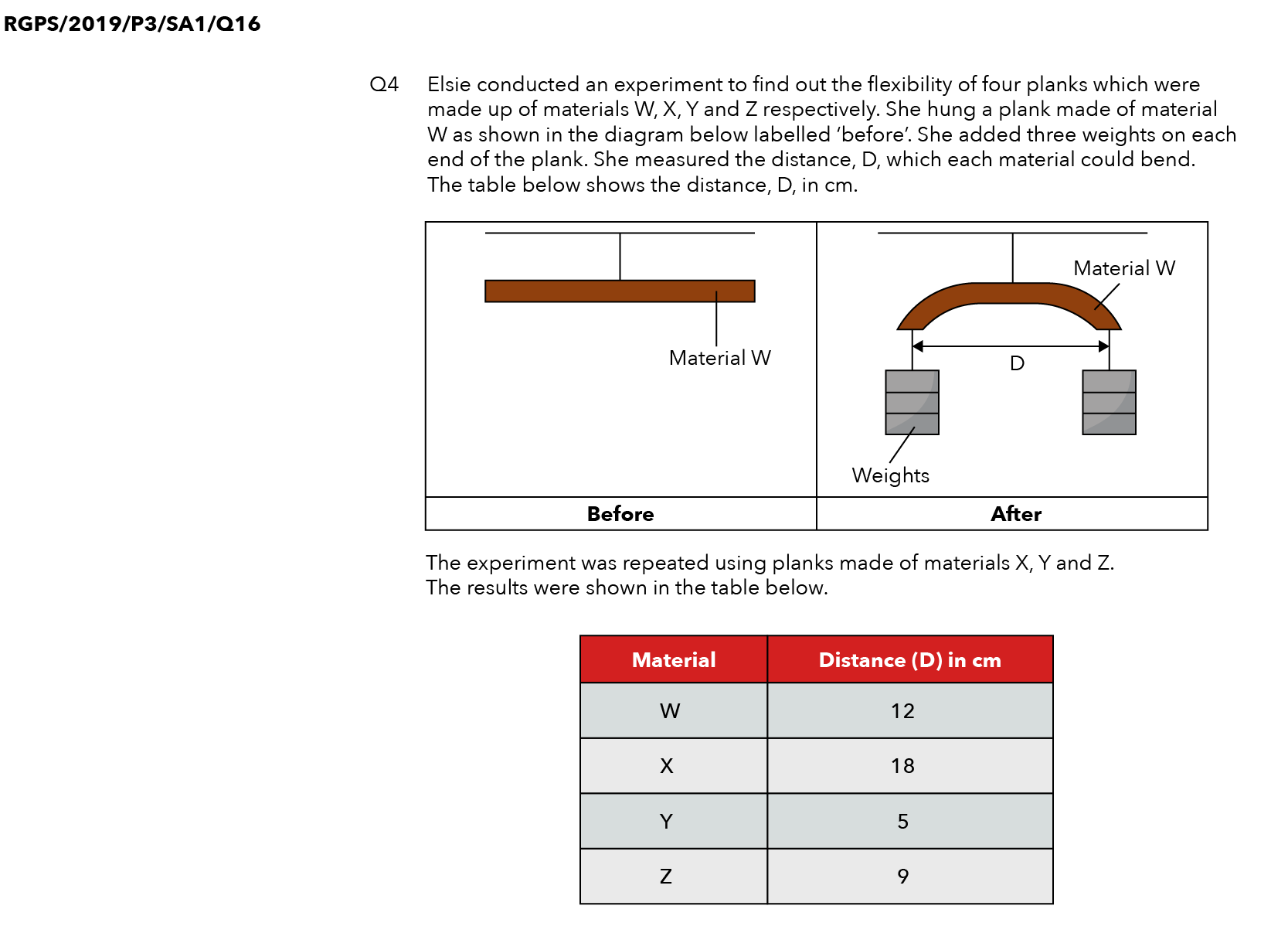Source: Raffles’ Girls Primary School (RGPS) – 2019 P3 SA1 Examination Paper [Q16]

## Question

Based on her results, which one of the following statements is the correct conclusion?

(1): Y is less flexible than Z.

(2): Z is more flexible than W.

(3): X is the most flexible material.

(4) Y is the least flexible material.

## Thought Process

Now, if you take a look at the 4 options above, the options talk about comparing the flexibility of all the materials, so let’s go and figure out which material is the most flexible.

Let me guess what some of you are thinking. Some of you think that because X has the greatest distance D, this means that X must be the most flexible. Now, is that necessarily true?

To answer this question, we need to figure out what distance D actually represents! So if you look at the diagram, distance D is the distance between the two ends of the material.

Now let me draw out another material in purple.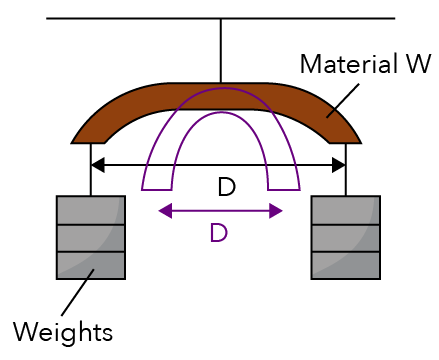Now what would be the distance D? Distance D will be represented by the distance between the two ends as shown above.

And if you look at this distance D, is this distance D bigger or smaller than the one in the diagram? This distance D is smaller!

In fact, when distance D is smaller, did the purple-coloured material manage to bend more or bend less?

This material was able to bend more!

And if the material could bend more, is this material more or less flexible?

This means that the material is more flexible.

So, is it necessarily true that the one with the greatest distance D will be the one that is the most flexible?

No! In fact, the smaller distance D is, the more the material is bent, which means the more flexible this material is.

So, if you want to find out which material is the most flexible, should we choose the one with the biggest distance D or the smallest?

We should choose the one with the smallest distance D!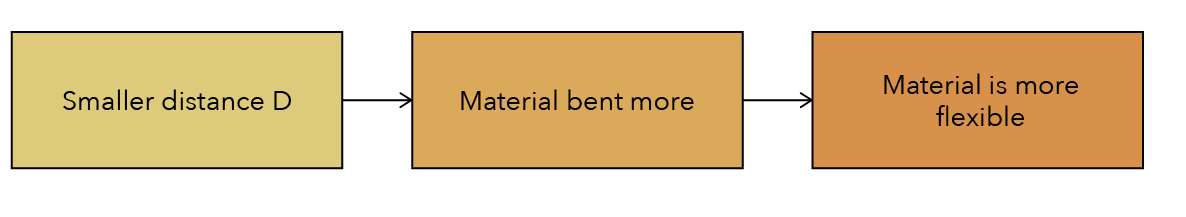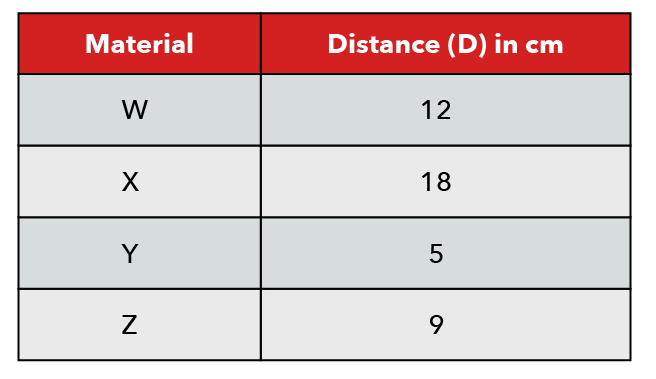Material Y has the smallest distance D so Y is the most flexible.

Since we need to compare the flexibility of the materials, let’s arrange them starting with the one that is the most flexible, all the way to the one that is the least flexible.

So we already know that Y is the most flexible. And this is followed by Z and then W.

And which one is the least flexible?

The least flexible material is the one with the largest distance D and that is X.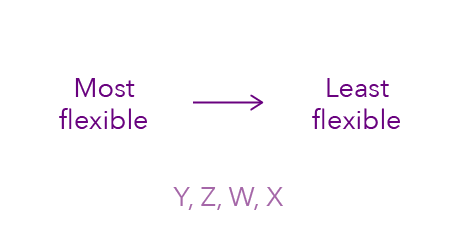So now that we have arranged them, we can take a look at the options!

### Option (1): Y Is Less Flexible Than Z.

Is this true? No! In fact, Y should be more flexible. We can cross out option (1).

### Option (2): Z Is More Flexible Than W.

Is this true? Definitely!

### Option (3): X Is The Most Flexible Material.

Is this true? No, in fact, X is the least flexible material! So, this option is out.

### Option (4) Y Is The Least Flexible Material.

Is this true? No! In fact, Y is the most flexible. So we can cross out option (4) too.